# Practical Data Science with Python Training

4.7
(4.7)

This course uses several graphs and visuals to explain all fundamental Data Science principles and their applications rather than complex mathematical calculations.

• Category : Data Science

Course Price : \$1595 Per Participant

## Course Description

You can learn practical data science with Python to gradually comprehend data science and its methods.

With the help of several examples, this course is created to aid in your understanding of data science in the most straightforward manner possible.

This course uses several graphs and visuals to explain all fundamental Data Science principles and their applications rather than complex mathematical calculations.

This course adopts a distinct methodology centered on providing straightforward illustrations of each Data Science technique's operation and builds incrementally on those illustrations to cover Data Science.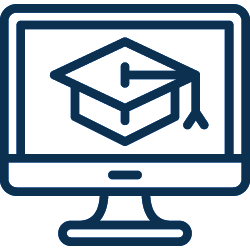500+

Courses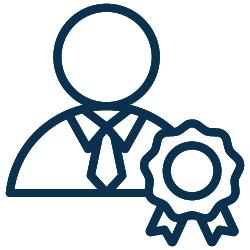20+

Years of Experience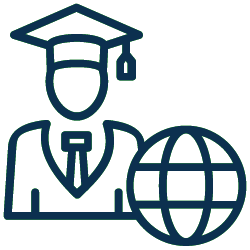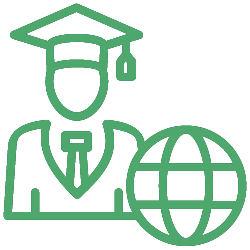95K+

Global Learners

## What you will learn

•Data collection, exploration, cleaning, munging, and manipulation
•Explore the foundations of machine learning.
•Use models like k-nearest Logistic regression and neighbors.
•Study the fundamentals of linear algebra, statistics, and probability and comprehend their applications in data science.

## Prerequisites

### Required

• Little programming experience is required.

### Recommended

• Python Programming - Introduction

## Who should attend this course?

• Beginners interested in Data Science but hesitant to begin due to their fear of complex math
• Beginners in the data science and computer science fields
• Experts in the social sciences and data
• Professors, lecturers, or tutors who want to simplify and improve how they teach their students new material
• Especially those who focus on Data Science are students and academics.

## Schedules

Oops! For this course, there are currently no public schedules available. Clicking on "Notify Me" will allow you to express your interest.

For dates, times, and location customization of this course, get in touch with us.

You can also speak with a learning consultant by calling 800-961-0337.

## Curriculum

• The Ascendance of Data
• What Is Data Science?
• Motivating Hypothetical: DataSciencester
• The Basics
• The Not-So-Basics
• matplotlib
• Bar Charts
• Line Charts
• Scatterplots
• Describing a Single Set of Data
• Correlation
• Some Other Correlational Caveats
• Correlation and Causation
• Dependence and Independence
• Conditional Probability
• Bayes's Theorem
• Random Variables
• Continuous Distributions
• The Normal Distribution
• The Central Limit Theorem
• For Further Exploration
• Modeling
• What Is Machine Learning?
• Overfitting and Underfitting
• Correctness
• Feature Extraction and Selection
• The Model
• Example: Favorite Languages
• The Curse of Dimensionality
• The Model
• Maximum Likelihood Estimation
• The Model
• Further Assumptions of the Least Squares Model
• Fitting the Model
• Interpreting the Model
• Goodness of Fit
• Digression: The Bootstrap
• Standard Errors of Regression Coefficients
• Regularization
• The Problem
• The Logistic Function
• Applying the Model
• Goodness of Fit
• Support Vector Machines
• ## Course Details

•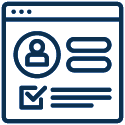Enrolled: 1493
•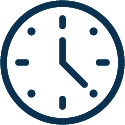Duration: 3 Days